Function Repository Resource:

# HypergraphNeighborhoodVolumes

Find the volumes of successively larger neighborhoods around nodes in a hypergraph

Contributed by: Stephen Wolfram
 ResourceFunction["HypergraphNeighborhoodVolumes"][graph] finds the count of neighbors within successive distances for each vertex in graph. ResourceFunction["HypergraphNeighborhoodVolumes"][graph,{v1,v2,…}] finds the counts for the vertices vi. ResourceFunction["HypergraphNeighborhoodVolumes"][graph,All,r] finds counts from all vertices out to maximum radius r. ResourceFunction["HypergraphNeighborhoodVolumes"][graph,{v1,v2,…},Automatic] finds counts only out to the minimum radius for any of the vertices vi. ResourceFunction["HypergraphNeighborhoodVolumes"][graph,"Random"→n,…] finds counts for n randomly chosen vertices.

## Details and Options

The hypergraph is assumed to be undirected.
ResourceFunction["HypergraphNeighborhoodVolumes"] uses ParallelMap.
The first element in each list is the zero-radius volume, followed by the distance one-radius volume, and so on.
The volume of the neighborhood of vertex v at radius r is the number of vertices connected to v within a distance of r hyperedges.

## Examples

### Basic Examples (1)

Neighbor counts for each vertex of a hypergraph:

 In:=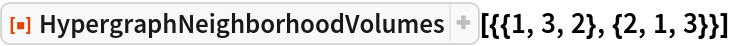Out=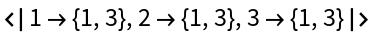### Scope (3)

Count neighbors for each vertex:

 In:=Out=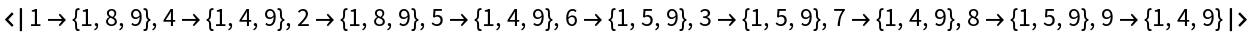Count neighbors for a subset of vertices:

 In:=Out=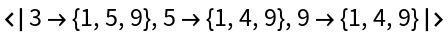Count neighbors for a random sampling of vertices:

 In:=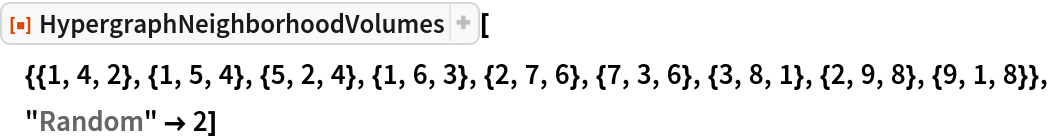Out=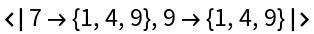## Version History

• 2.0.0 – 14 April 2020
• 1.0.0 – 18 February 2020